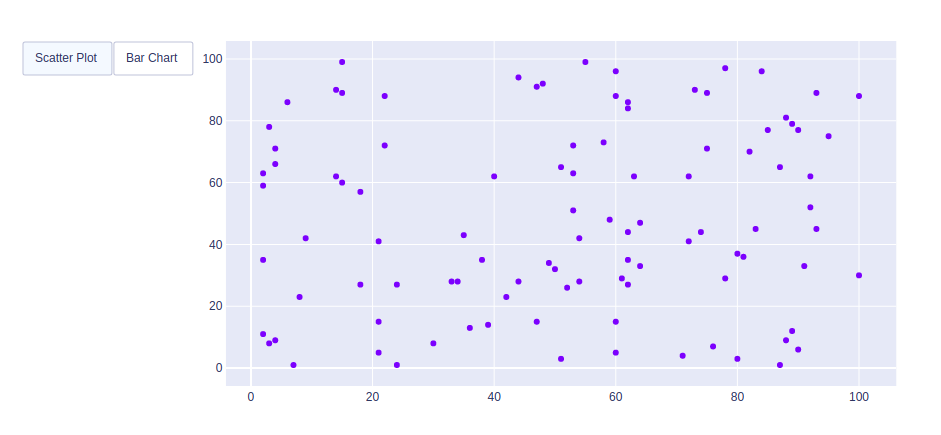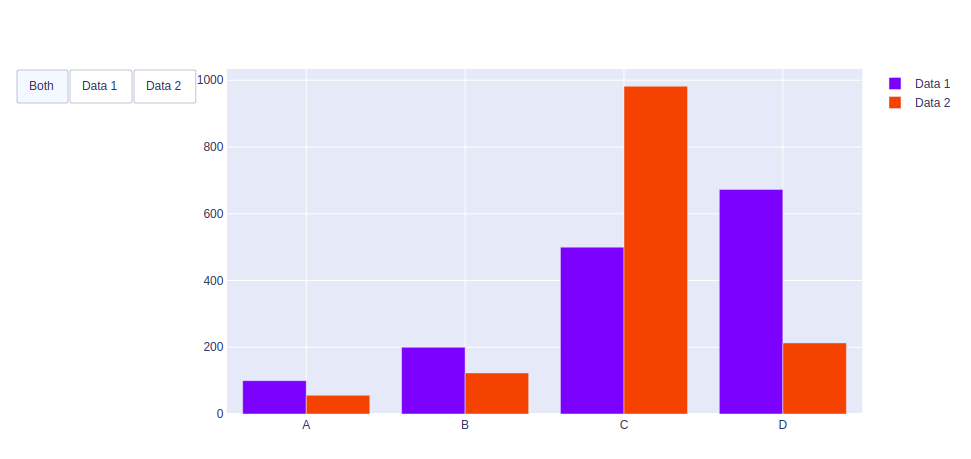GFG App
Open AppBrowser
Continue

# How to make Custom Buttons in Plotly?

A Plotly is a Python library that is used to design graphs, especially interactive graphs. It can plot various graphs and charts like histogram, barplot, boxplot, spreadplot, and many more. It is mainly used in data analysis as well as financial analysis. plotly is an interactive visualization library.

## Making Custom Buttons

In plotly, actions custom Buttons are used to quickly make actions directly from a record. Custom Buttons can be added to page layouts in CRM, Marketing, and Custom Apps. There are 4 possible methods that can be applied in custom buttons:

• restyle: modify data or data attributes
• relayout: modify layout attributes
• update: modify data and layout attributes
• animate: start or pause an animation

Example 1: Using Restyle Method

## Python3

 `import` `plotly.graph_objects as px ` `import` `numpy as np ` ` `  ` `  `# creating random data through randomint ` `# function of numpy.random ` `np.random.seed(``42``) ` ` `  `random_x ``=` `np.random.randint(``1``, ``101``, ``100``) ` `random_y ``=` `np.random.randint(``1``, ``101``, ``100``) ` ` `  `plot ``=` `px.Figure(data``=``[px.Scatter( ` `    ``x``=``random_x, ` `    ``y``=``random_y, ` `    ``mode``=``'markers'``,) ` `]) ` ` `  `# Add dropdown ` `plot.update_layout( ` `    ``updatemenus``=``[ ` `        ``dict``( ` `            ``type``=``"buttons"``, ` `            ``direction``=``"left"``, ` `            ``buttons``=``list``([ ` `                ``dict``( ` `                    ``args``=``[``"type"``, ``"scatter"``], ` `                    ``label``=``"Scatter Plot"``, ` `                    ``method``=``"restyle"` `                ``), ` `                ``dict``( ` `                    ``args``=``[``"type"``, ``"bar"``], ` `                    ``label``=``"Bar Chart"``, ` `                    ``method``=``"restyle"` `                ``) ` `            ``]), ` `        ``), ` `    ``] ` `) ` ` `  `plot.show() `

Output:Example 2: Using Update method

## Python3

 `import` `plotly.graph_objects as px ` `import` `numpy ` ` `  ` `  `# creating random data through randomint ` `# function of numpy.random ` `np.random.seed(``42``) ` ` `  `random_x ``=` `np.random.randint(``1``, ``101``, ``100``) ` `random_y ``=` `np.random.randint(``1``, ``101``, ``100``) ` ` `  `x ``=` `[``'A'``, ``'B'``, ``'C'``, ``'D'``] ` ` `  `plot ``=` `px.Figure(data``=``[go.Bar( ` `    ``name``=``'Data 1'``, ` `    ``x``=``x, ` `    ``y``=``[``100``, ``200``, ``500``, ``673``] ` `), ` `    ``go.Bar( ` `    ``name``=``'Data 2'``, ` `    ``x``=``x, ` `    ``y``=``[``56``, ``123``, ``982``, ``213``] ` `) ` `]) ` ` `  ` `  `# Add dropdown ` `plot.update_layout( ` `    ``updatemenus``=``[ ` `        ``dict``( ` `            ``type``=``"buttons"``, ` `            ``direction``=``"left"``, ` `            ``buttons``=``list``([ ` `                ``dict``(label``=``"Both"``, ` `                     ``method``=``"update"``, ` `                     ``args``=``[{``"visible"``: [``True``, ``True``]}, ` `                           ``{``"title"``: ``"Both"``}]), ` `                ``dict``(label``=``"Data 1"``, ` `                     ``method``=``"update"``, ` `                     ``args``=``[{``"visible"``: [``True``, ``False``]}, ` `                           ``{``"title"``: ``"Data 1"``, ` `                            ``}]), ` `                ``dict``(label``=``"Data 2"``, ` `                     ``method``=``"update"``, ` `                     ``args``=``[{``"visible"``: [``False``, ``True``]}, ` `                           ``{``"title"``: ``"Data 2"``, ` `                            ``}]), ` `            ``]), ` `        ``) ` `    ``]) ` ` `  `plot.show() `

Output:My Personal Notes arrow_drop_up